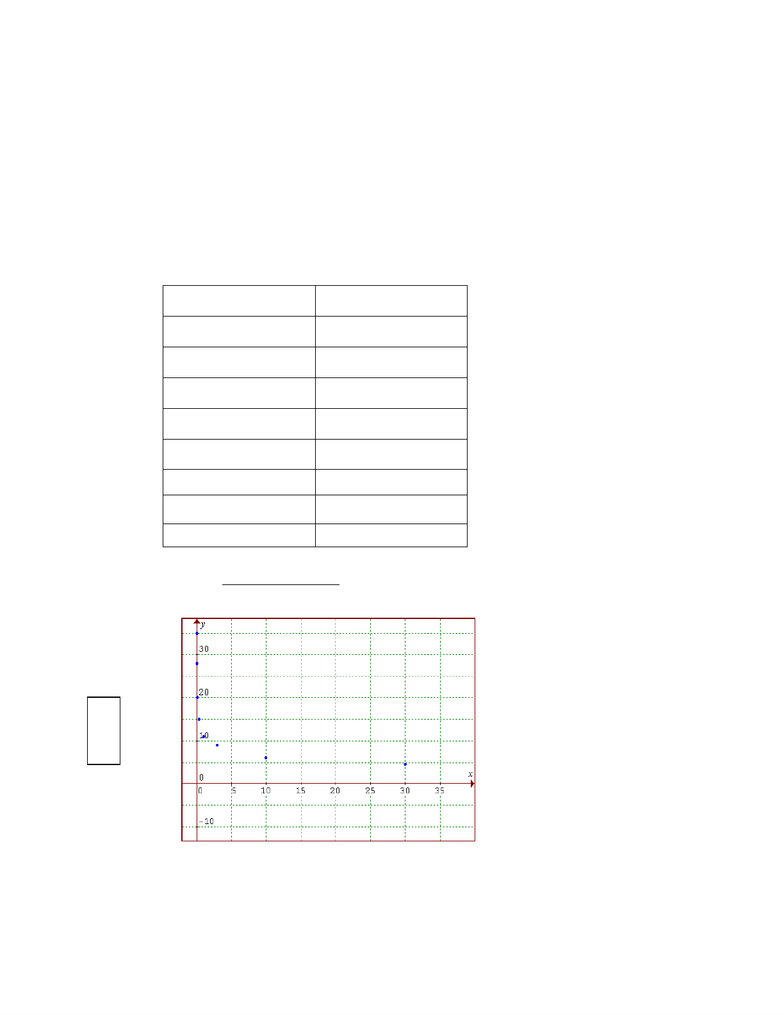# math portfolio 2.docx

9 views13 pages
School
Department
Course
ProfessorAim: In this task you will develop model functions representing the tolerance of human beings
to G-forces over time.
Introduction and Background
The resulting acceleration on an object as a result of several different forces acting on it
is referred to as “G-force”. The force is in relation to gravity, in that it is the force of gravity
acting on a celestial body. A G-force is equal to twice the force of gravity 2g(“2gees”).
According to Astronauts there are different forms of G-forces. “Eye balls-in” refers to
forward acceleration, where the body is pushed backwards and backwards acceleration is
referred to as “eyeballs-out”.
It’s been proven human’s are able to withstand forward acceleration rather than
backwards acceleration. Studies showed that humans are able to withstand 17g eyeballs in
compared to 12 g eyeballs out, and were able to do so for a long period time still maintaining
consciousness. Some examples are roller coaster rides which expose humans to these G-forces
but to small samples and in very short time periods.
Unlock document

This preview shows pages 1-3 of the document.
Unlock all 13 pages and 3 million more documents.+Gx(g)
The following table illustrates the tolerance beings to horizontal G-force. The notation “+Gx”
represents a positive acceleration in the horizontal direction i.e. eyeballs-in, so that a force of
+Gx of 20 means a forward accelerating of 20 (which humans can tolerate for 0.1 minutes).
Horizontal G-Force
Time (minutes)
Time (mins)
+Gx (g)
0.01
35
0.03
28
0.1
20
0.3
15
1
11
3
9
10
6
30
4.5
Unlock document

This preview shows pages 1-3 of the document.
Unlock all 13 pages and 3 million more documents.The graphing program I used was Geogebra. The program allowed me to create the
graph and also manipulate the x and y numbers to match that of the chart above. I was also
able to manipulate the image so I could capture an appropriate shot. In the graph, my x values
are represented by the time values. The time is represented by the domain of     
where x  because the value of time can not equal 0. The value of “y” is represented by the
horizontal G-force     . The graph appears to be decreasing in relation to the
horizontal G-force in relation to an increase in time. This suggests that human beings can
tolerate a significantly less horizontal G-force for an extended period of time. This will only lead
to assume this trend will continue so long as the graph continues
To plot the ln (x) I used the program graphmatica, it was able to effectively plot the graph
y= ln (x) and y= -ln (x). The reason I chose the function y= -ln (x) was because it would be
reflected through the x-axis and this more accurately reflect the graph of the “tolerance of
human beings to horizontal G-force.” As well the asymptote of    would still be reflected in
this graph. The graph in red represents the function y= ln(x) and the graph in white represents
the function y= -ln(x).
Unlock document

This preview shows pages 1-3 of the document.
Unlock all 13 pages and 3 million more documents.

# Get access

\$10 USD/m
Billed \$120 USD annually
Homework Help
Class Notes
Textbook Notes